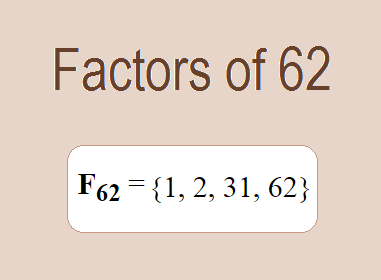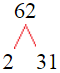# Factors of 62The factors of 62 are 1, 2, 31, and 62 i.e. F62 = {1, 2, 31, 62}. The factors of 62 are all the numbers that can divide 62 without leaving a remainder.

We can check if these numbers are factors of 62 by dividing 62 by each of them. If the result is a whole number, then the number is a factor of 62. Let's do this for each of the numbers listed above:

·        1 is a factor of 62 because 62 divided by 1 is 62.

·        2 is a factor of 62 because 62 divided by 2 is 31.

·        31 is a factor of 62 because 62 divided by 31 is 2.

·        62 is a factor of 62 because 62 divided by 62 is 1.

## How to Find Factors of 62?

1 and the number itself are the factors of every number. So, 1 and 62 are two factors of 62. To find the other factors of 62, we can start by dividing 62 by the numbers between 1 and 62. If we divide 62 by 2, we get a remainder of 0. Therefore, 2 is a factor of 62. If we divide 62 by 3, we get a remainder of 2. Therefore, 3 is not a factor of 62.

Next, we can check if 4 is a factor of 62. If we divide 62 by 4, we get a remainder of 2. Therefore, 4 is not a factor of 62. We can continue this process for all the possible factors of 62.

Through this process, we can find that the factors of 62 are 1, 2, 31, and 62. These are the only numbers that can divide 62 without leaving a remainder.

********************

********************

## Properties of the Factors of 62

The factors of 62 have some interesting properties. One of the properties is that the sum of the factors of 62 is equal to 96. We can see this by adding all the factors of 62 together:

1 + 2 + 31 + 62 = 96

Another property of the factors of 62 is that the prime factors of 62 are 2 and 31 only.

## Applications of the Factors of 62

The factors of 62 have several applications in mathematics. One of the applications is in finding the highest common factor (HCF) of two or more numbers. The HCF is the largest factor that two or more numbers have in common. For example, to find the HCF of 62 and 31, we need to find the factors of both numbers and identify the largest factor they have in common. The factors of 62 are 1, 2, 31, and 62. The factors of 31 are 1, and 31. The largest factor that they have in common is 31. Therefore, the HCF of 62 and 31 is 31.

Another application of the factors of 62 is in prime factorization. Prime factorization is the process of expressing a number as the product of its prime factors. The prime factors of 62 are 2 and 31 since these are the only prime numbers that can divide 62 without leaving a remainder. Therefore, we can express 62 as:

62 = 2 × 31

We can do prime factorization by division and factor tree method also. Here is the prime factorization of 62 by division method,62 = 2 × 31

Here is the prime factorization of 62 by the factor tree method,62 = 2 × 31

## Conclusion

The factors of 62 are the numbers that can divide 62 without leaving a remainder. The factors of 62 are 1, 2, 31, and 62. The factors of 62 have some interesting properties, such as having a sum of 96. The factors of 62 have several applications in mathematics, such as finding the highest common factor and prime factorization.